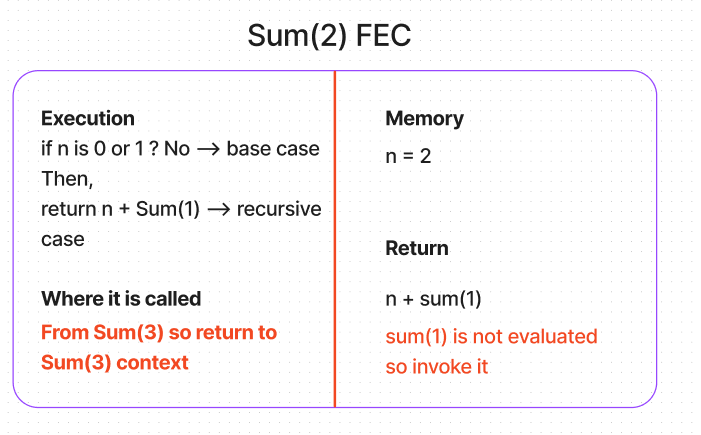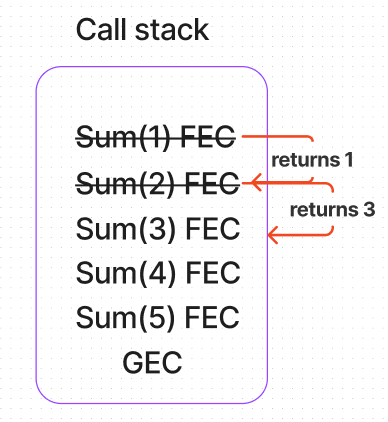top of page

# Understanding Recursion in Javascript.

We need to understand the call stack in javascript to understand the whole picture of how recursion works.

In-depth explanation here.

##### TL;DR:
• Every programmer deals with data.

• We either create data or give functionality to them(basically working on them).

• You need memory to store data.

• You need to access the data from memory --> Birth of variables.

• We do operations or perform logical operations on data.

• We can store logic --> Birth of functions.

• We can use the logic whenever we need them --> Birth of function invocation or function call.

• Logics are performed upon data; we need memory to store the data and variables to access them.

• Logics can be inside or outside the functions --> Birth of functional and global execution context.

• Execution context is the packaging of logic and their needed memory --> in a super simplified sense.

• Logic + memory = Execution context, and logic can be functional or global, hence the Functional Execution context(FEC) or Global Execution context(GEC).• Every program starts with creating GEC, and whenever it comes across function invocation --> it creates FEC on top of GEC.

• Function invocation can happen inside another function(Higher order function). Therefore new FEC sits on top of the older or Higher Order FEC.

• This arrangement of Execution Contexts with GEC at its base is called Call Stack.

##### What is Recursion?

Suppose I start from the definition and lead you to examples of recursion, it would be the most inappropriate way to learn recursion(in fact, any concept). By the way, it is not understanding but defining.

But the word 'Recursion' has its origin in Latin,

The etymology of recursion is utterly straightforward. It is from the Latin 'recursionem' meaning “a running backward, return.” The root is the same for the better-known verb “to recur.”

Note: Running backward or return

We try moving from known knowledge of recursion,

If you encounter recursion anywhere in the code, you will likely see the function calling itself.

Wait! what is the function calling itself, by the way?

What would happen to the call stack if the functions call themselves inside it?

```function CallingItself() {
CallingItself()
}

CallingItself(); // Function invocation```

If you run the above code, you would get,

`RangeError: Maximum call stack size exceeded`

The call stack builds upon the same function when the function calls itself.This error occurs because there is no end to the "CallingItself" function. It keeps calling itself until the max stack size is reached, and the compiler throws an error.

So somebody says recursion means function calling itself --> It is not fully correct or they are defining partially.

So to use recursion,

We need to know where to stop.

Where to continue calling itself.

These are called Base case and Recursive case in programming jargon.

Base case = Stop function calling and return the value.
Recursive case = return function calling.

So, where does the function call itself?

If the base case tells when to stop calling itself, the naturally recursive case contains a function calling itself.

How to connect the base case and recursive case?

• If the recursive case keeps on running --> stack overflow error.

• If only base case --> we are not using recursion itself.

• Finding a point to connect...

• The recursive case keeps on running (calling the same function) until it reaches the base case. That's the first principle.

• So the whole function lands on the base case at one point and returns the value.

• Here function and its definition won't change.

• We need to find something that changes --> Yes, it is parameters in the function.

Parameters keep changing when the function calls itself in a recursive case until it lands on the base case.

Note: I am not beating around the bush, and this is just decluttering.

##### How the call stack appears?

Let us give one example so that we can understand and connect all the dots.

We will do a sum of numbers.

Like if I give 5 as a parameter --> it should give 5 + 4 + 3+ 2 +1 + 0 = 15.

For 8 --> 8 + 7 + 6 + 5 + 4 + 3 + 2 +1 + 0 = 36.Likewise!

```function Sum(n) {
// Base case
if(n === 0) return 0 // This base case takes care when parameter is 0
if(n === 1) return 1 // This base case returns 1

// Recursive case
else {
return n + sum(n-1) // Function callng itself with parameter change
}
}
const output = Sum(5) // 15```

We will FEC and Call stack here,

Points to remember:

• Execution context contains memory, execution, where it is called, and return value.

• Function can't return any value until it evaluates the full expression(n + sum(n-1)). Here sum(n-1) is not evaluated. Therefore it keeps on calling itself.

• Any function returns value to the context where it is called.When the compiler sees Sum(5) invocation, it adds Sum(5) FEC on top of GEC.

Now the FEC Sum(5) is created.• Since Sum(5) is not returned, it is not removed from the call stack.

• Also, it calls Sum(4) in the return statement.

• It adds Sum(4) FEC into the stack.

• It must be above Sum(5) FEC because it is not removed.The same process repeats,• Since Sum(4) is not returned, it is not removed from the call stack.

• Also, it calls Sum(3) in the return statement.

• It adds Sum(3) FEC into the stack.

• It must be above Sum(4) FEC because it is not removed.Again,• Since Sum(3) is not returned, it is not removed from the call stack.

• Also, it calls Sum(2) in the return statement.

• It adds Sum(2) FEC into the stack.

• It must be above Sum(3) FEC because it is not removed.Again,• Since Sum(2) is not returned,it is not removed from the call stack.

• Also, it calls Sum(1) in the return statement.

• It adds Sum(1) FEC into the stack.

• It must be above Sum(2) FEC because it is not removed.##### What happens to the call stack now?
It pops Sum(1) from the call stack by returning the value to Sum(2) execution context.Let's look at Sum(2) FEC,So call stack is,If we follow similar steps, we land up inHence the output will be in 15. Yes, we finally got it.

So Recursion = Building call stack until base case + return values from top to GEC.

After all, recursion is running backward or return.

The etymology of recursion is utterly straightforward. It is from the Latin 'recursionem' meaning “a running backward, return.” The root is the same for the better-known verb “to recur.”

## Recent Posts

See All

As there are many programming languages, there are many programming paradigms. Programming paradigm = style of programming = set of guidelines to solve the problem. So, many programming languages evol

bottom of page